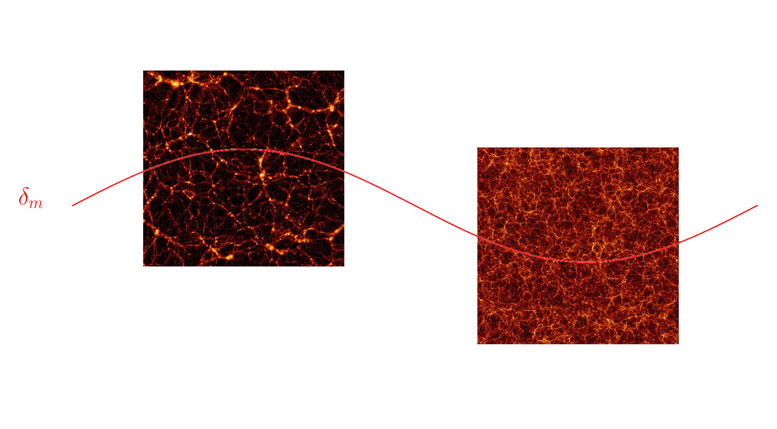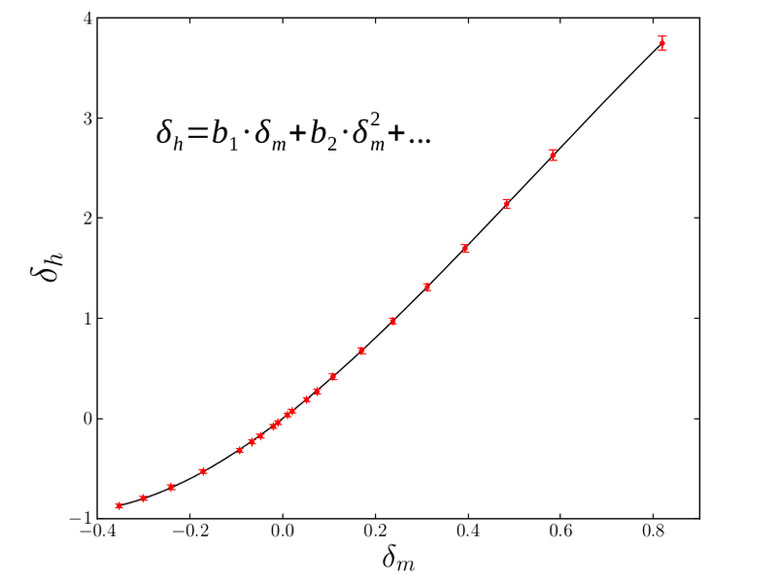# Researchers Use Separate Universe Simulations to Study the Clustering of Dark MatterFigure 1: Schematic representation of the separate universe idea. The red line represents a long wavelength matter density perturbation. The two panels show results of separate universe simulations in initially overdense (left) and underdense regions (right). The color indicates the matter density with lighter regions being denser.

Using the separate universe simulations technique, researchers reveal some of the most precise measurements of the local bias – confirming the known trend that more massive halos are more biased than smaller halos.

In the standard cosmological model, dark matter makes up roughly 25 % of the total energy budget of the Universe. However it cannot be observed directly, since it does not emit light. Understanding the way dark matter clusters together and forms structures is of crucial importance since it would help our understanding of the observed spatial distribution of galaxies (which should closely follow the dark matter distribution) and link this with early-Universe physics and the origin of initial perturbations. In this context, researchers at MPA and in other institutions worldwide came up with a new way of simulating the impact of large-scale primordial perturbations on the abundance of structures observed at late times, the so-called separate universe simulations. Using this technique, the MPA researchers recently obtained some of the most precise measurements of the local bias, confirming the known trend that more massive halos are more biased than smaller halos.

Dark matter groups itself in various structures to create what is called the cosmic web (see Figure 1). One of the most important components are the so-called halos, which simply represent regions where dark matter has accumulated. The abundance and distribution of these halos is strongly dependent on the distribution of dark matter: one could also say that the halo distribution is biased with respect to the dark matter distribution. Understanding this bias and what physical effects affect it is of crucial importance for the statistical description of the halo distribution. This, in turn, is very important as the current paradigm states that cosmological tracers (such as galaxies or galaxy clusters) reside preferentially in dark matter halos.

To study dark matter clustering, physicists traditionally run so-called “N-body” numerical simulations. In their simplest form, these simulations follow a set of particles in a box from an initial distribution to some later time, using Newtonian physics to describe the evolution and our knowledge of dark matter properties. Here the term “particle” is a substitute for “mass element”, which are normally on the order of a few million or even billion solar masses, as we are not able to achieve infinite mass resolution. In order to cover a wide range of scales, these simulations must both have a large number of particles (billions) and be of largest possible volume (up to a few Gpc on a side) which means that they quickly become costly computationally.

Separate universe simulations

The main idea behind the separate universe simulations is that a patch of the Universe, which has a different matter density, is treated as a separate universe. Indeed, it can be shown that applying an overall uniform change to the matter density in the simulation (i.e. adding a perturbation with an infinite wavelength) is equivalent to running the simulation with different cosmological parameters (for example going from flat to curved geometry). Hence, it is possible to divide a costly big simulation into smaller ones, where each has a different matter density and correctly adjusted other cosmological parameters. Thus, the dependence of e.g. the density of halos on the matter density can be studied in a clean way.

This technique does not only make the running of simulations easier, it makes it also possible to measure the impact of large-scale perturbations on smaller scales where halo and galaxy formation takes place. Since the overall matter density is now a parameter that can be chosen independently, it is possible to measure the dependence of structure formation on this parameter solely – unlike in traditional N-body simulations where a mixing of scales is unavoidable. Hence it is a fast and easy way to make precise measurements of quantities depending on the matter density.Figure 2: The dependence of halo density (δh) on the matter density (δm) in separate universes. Red points show results from simulations and the black line presents the fit of a polynomial to extract the local bias parameters.

A measure of clustering: halo bias

One of these quantities is the density of dark matter halos found in a simulation. As mentioned above, the bias is the statistical quantity linking the halo density to the matter density. While there are many different bias parameters reflecting the various physical effects entering structure formation, the most well-studied bias parameter on large scales is the so-called local bias. This local bias simply relates the halo density to the matter density at each location in the simulation.

Separate universe simulations provide a perfect framework to obtain precise measurements of this quantity, as one simply needs to run several simulations with various values for the matter density and to measure the final density of dark matter halos in each of them. The obtained relation between matter and halo density then gives the local bias parameter as the proportionality constant between these two quantities, as is shown in Figure 2.

Clearly, simulations with higher initial matter density lead to higher halo density at later times, as can also be seen in Figure 1. Using this technique, researchers at MPA recently obtained some of the most precise measurements of the local bias, confirming the known trend that more massive halos (which are also less common) are more biased than smaller halos.

References:

• “Separate Universe Simulations” by Christian Wagner, Fabian Schmidt, Chi-Ting Chiang and Eiichiro Komatsu, 31 December 2014, MNRAS Letters.
DOI: 10.1093/mnrasl/slu187
arXiv
• “Precision measurement of the local bias of dark matter halos” by Titouan Lazeyras, Christian Wagner, Tobias Baldauf and Fabian Schmidt, 8 February 2016, Journal of Cosmology and Astroparticle Physics.
DOI: 10.1088/1475-7516/2016/02/018
arXiv